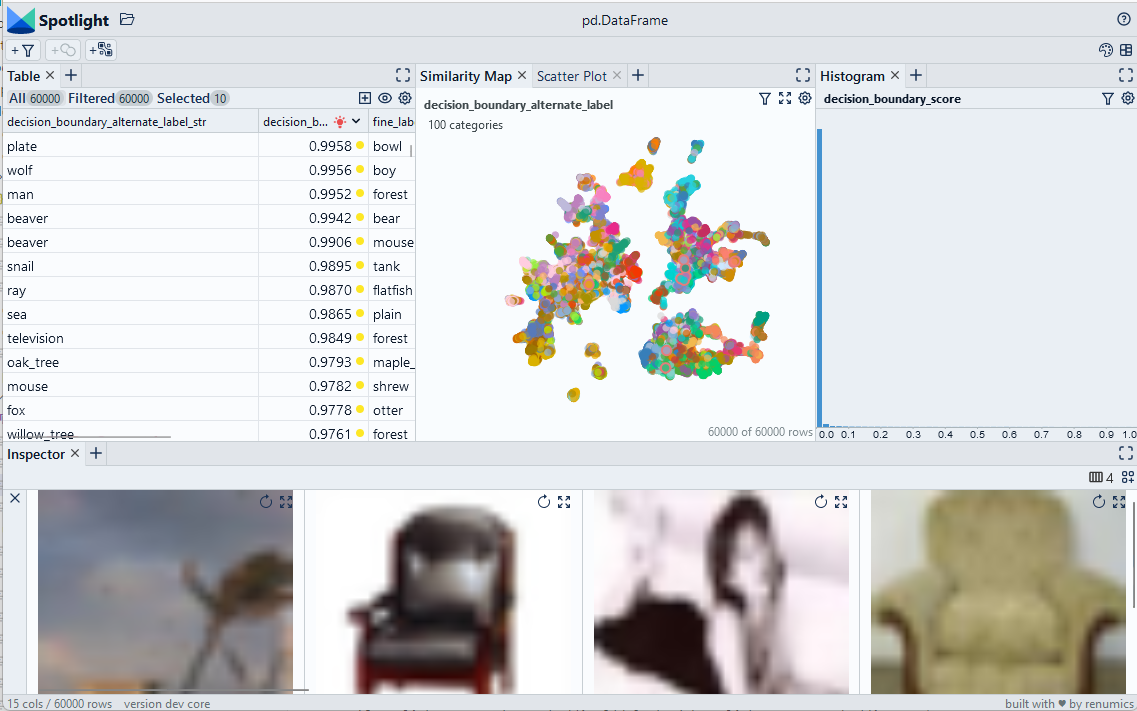🎉 We released Spotlight 1.4.0 check it out

Version: Next

# Detect decision boundaries based on certainty ratios

We use certainty ratios to compute a decision boundary score.

Use Chrome to run Spotlight in Colab. Due to Colab restrictions (e.g. no websocket support), the performance is limited. Run the notebook locally for the full Spotlight experience.## Imports and play as copy-n-paste functions​

# Install dependencies
``#@title Install required packages with PIP!pip install renumics-spotlight datasets``
# Play as copy-n-paste functions
``import datasetsimport numpy as npimport pandas as pdfrom renumics import spotlightimport requestsdef boundary_score(df, probabilities_name='probabilities'):    def compute_score(probs):        indices=np.argsort(probs)[::-1]        score = [indices, indices, probs[indices],probs[indices] ]        return score    df_out=pd.DataFrame()    temp_scores=[compute_score(x) for x in df[probabilities_name]]    df_out['decision_boundary_score']=[x/x for x in temp_scores]    df_out['decision_boundary_alternate_label']=[x for x in temp_scores]    return df_out``

## Step-by-step example on CIFAR-100​

### Load CIFAR-100 from Huggingface hub and convert it to Pandas dataframe​

``dataset = datasets.load_dataset("renumics/cifar100-enriched", split="train")df = dataset.to_pandas()``

### Compute decision boundary score and alternate label​

``f_boundary = boundary_score(df)df = pd.concat([df, df_boundary], axis=1)df['decision_boundary_alternate_label_str']=[dataset.features["fine_label"].int2str(x) for x in df['decision_boundary_alternate_label']]``

### Inspect decision boundary with Spotlight​

``df_show = df.drop(columns=['embedding', 'probabilities'])layout_url = "https://raw.githubusercontent.com/Renumics/spotlight/playbook_initial_draft/playbook/rookie/decision_boundary_layout.json"response = requests.get(layout_url)layout = spotlight.layout.nodes.Layout(**json.loads(response.text))spotlight.show(df_show, dtype={"image": spotlight.Image, "embedding_reduced": spotlight.Embedding}, layout=layout)``